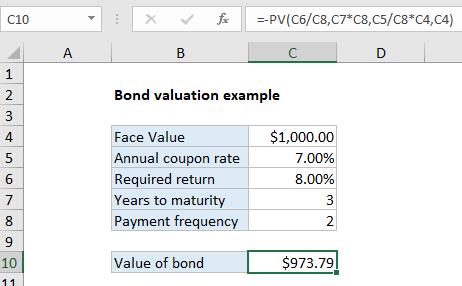# Bond valuation example in Excel

This tutorial show how to calculate the value of a bond on the issue date using the PV function.## Explanation

In the example shown, the formula in C10 is:

`=-PV(C6/C8,C7*C8,C5/C8*C4,C4)`

Note: This example assumes that today is the issue date, so the next payment will occur in exactly six months. See note below on finding the value of a bond on any date.

Worked Example:   PMT, RATE, NPER, PV and FV Financial Functions in Excel

### How this formula works

In the example shown, we have a 3-year bond with a face value of \$1,000. The coupon rate is 7% so the bond will pay 7% of the \$1,000 face value in interest every year, or \$70. However, because interest is paid semiannually in two equal payments, there will be 6 coupon payments of \$35 each. The \$1,000 will be returned at maturity. Finally, the required rate of return (discount rate) is assumed to be 8%.

Worked Example:   How to calculate present value of annuity in excel

The value of an asset is the present value of its cash flows. In this example we use the PV function to calculate the present value of the 6 equal payments plus the \$1000 repayment that occurs when the bond reaches maturity. The PV function is configured as follows:

`=-PV(C6/C8,C7*C8,C5/C8*C4,C4)`

The arguments provided to PV are as follows:

Worked Example:   PV function: Description, Usage, Syntax, Examples and Explanation

rate – C6/C8 = 8%/2 = 4%

nper – C7*C8 = 3*2 = 6

pmt – C5/C8*C4 = 7%/2*1000 = 35

fv – 1000

The PV function returns -973.79. To get positive dollars, we use a negative sign before the PV function to get final result of \$973.79